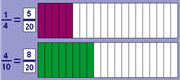### Addition and Subtraction of Fractions

 Addition and Subtraction of Fractions Menu of items to help you with this moduleStandards:5.NF.15.NF.2 Vocabulary:denominatorbenchmark fractionequivalent fractionVideos for each lesson can be found at this site.  Click on the correct module tab along the top, then click on the lesson.Practice Sites and Games- Fractions:Topic A: Equivalent Fractions Lesson 1:  Equivalent FractionsVideo- Equivalent Fractions     Lesson 2:  Equivalent Fractions with sums of FractionsVideo     Topic B:  Making Units Pictorially Lesson 3:  Adding Fractions with Unlike Denominators Lesson 4:  Adding fractions with sums between 1 and 2      LZ2659  Add fractions using an area model Lesson 5:  Subtract fractions using an area model     LZ2624  Subtract fractions Lesson 6:  Subtract fractions from numbers between 1 and 2Lesson 7:  Solve two-step word problems       Topic C:  Making Like Units NumericallyLesson 8: Add and Subtract fractions using a number lineLesson 9:  Add fractions making like units numericallyLesson 10:  Adding Larger FractionsLesson 11:  Subtract fractions using like units numericallyLesson 12:  Subtracting fractionsTopic D:  Further ApplicationsLesson 13:  Using Benchmark fractions    LZ1321   Compare fractions to benchmark of 1/2 using a number line    LZ1322  Compare fractions to benchmark of 1/2 using area models    LZ848    Compare fractions to a benchmark of 1 using a number line    LZ849    Compare fractions to a benchmark of 1 using area modelsLesson 14:  Problem Solving    Video HW helpLesson 15:  More Problem Solving     Video HW help    Video on lessonLesson 16:  Parts of a Whole    Video-- Problem Set    Video- Lesson Explanation